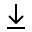MathBin Integer Member

An integer is a number that can be written without a fractional component.

Bins
1. Binomial Coefficient (MMA)
2. Factorial (JS)
3. Fibonacci Number (JS)
4. Greatest Common Divisor (MMA)
5. Least Common Multiple (MMA)
6. Prime Factorization (MMA and JS)